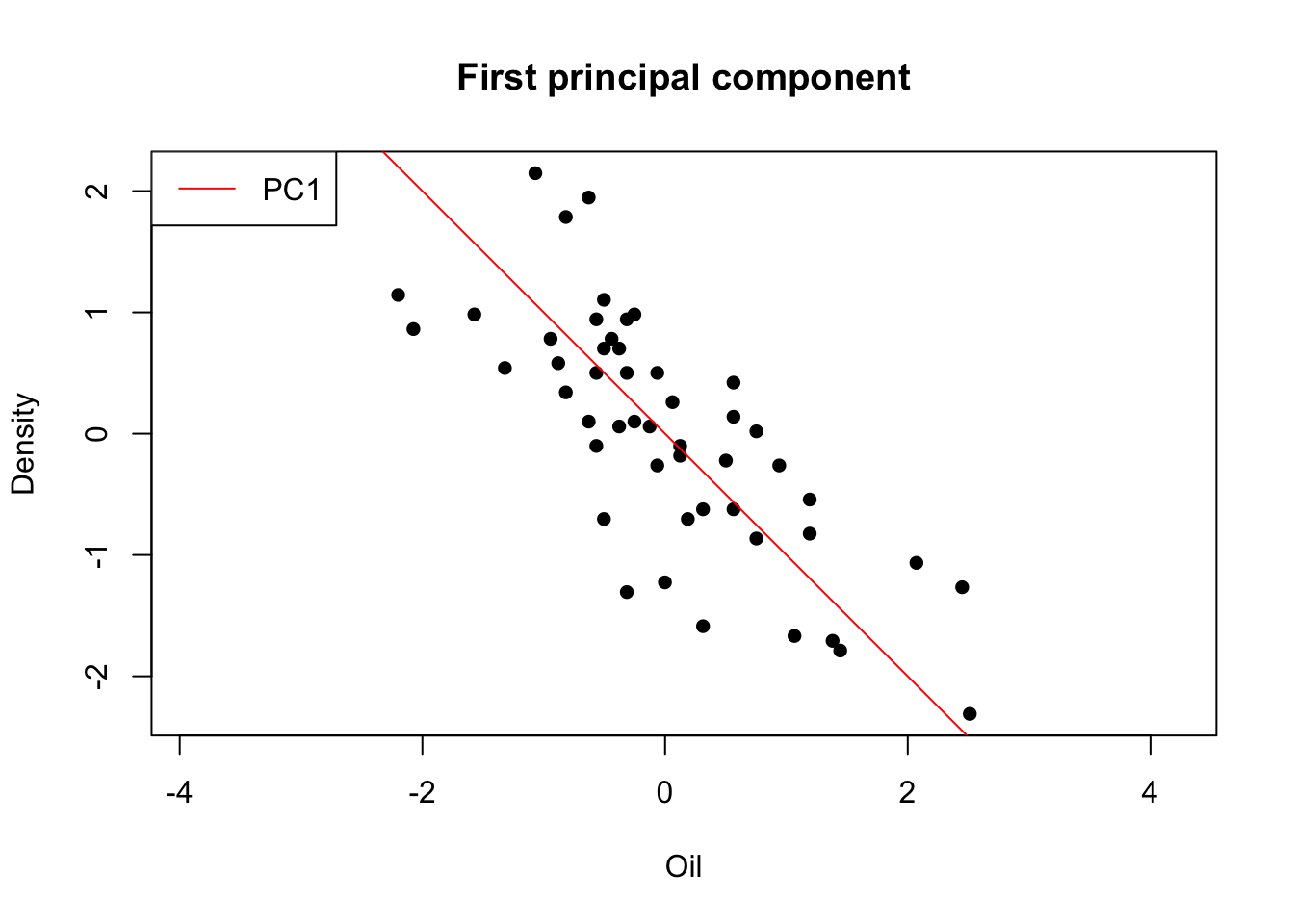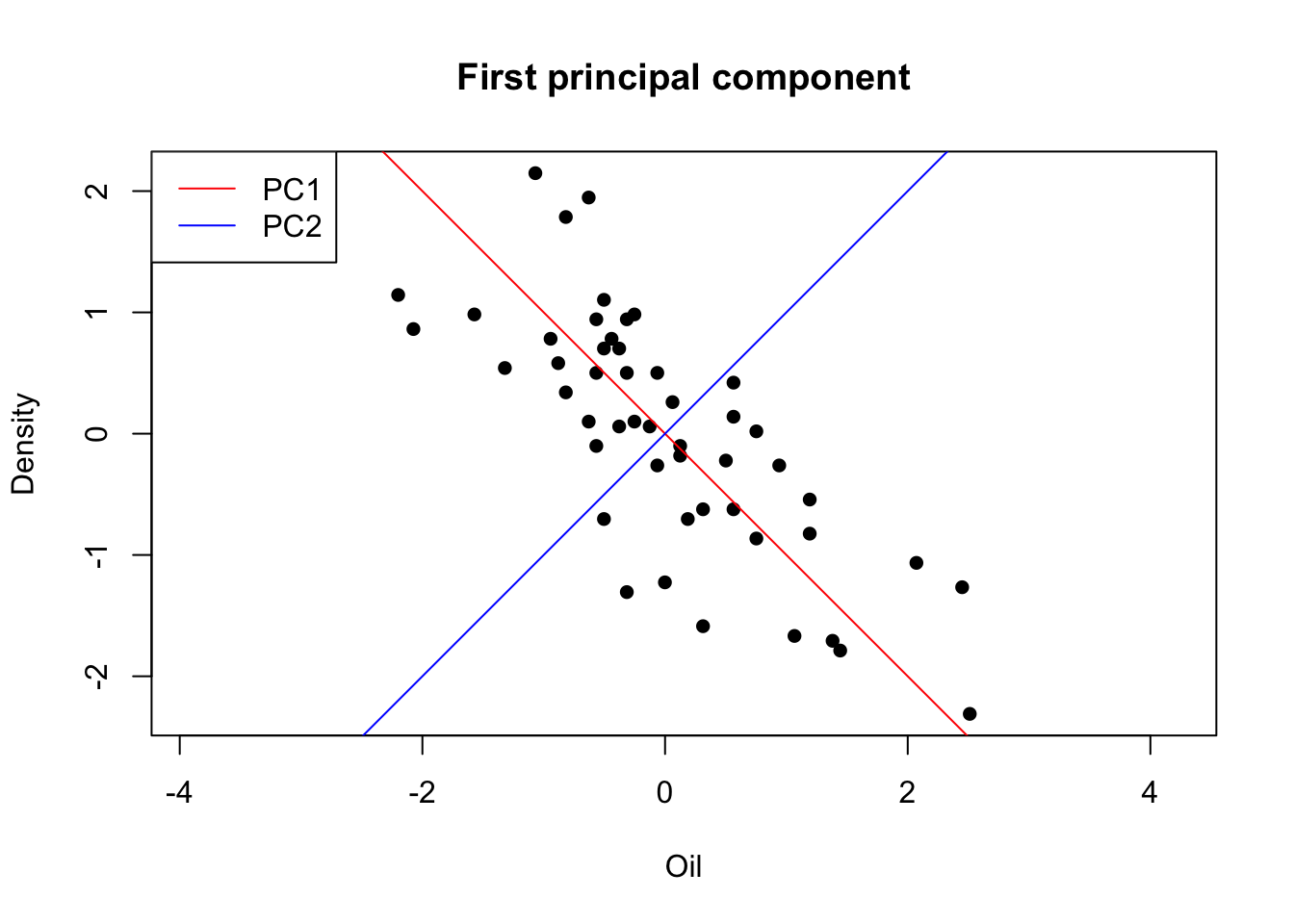Suppose we have $$n$$ measurements on a vector $$\mathbf x$$ of $$d$$ features, and we wish to reduce the dimension from $$d$$ to $$k$$, where $$k$$ is typically much smaller than $$d$$. PCA does this by finding linear combinations, $$\phi_1 \mathbf x, \phi_2 \mathbf x, ..., \phi_k \mathbf x$$, called principal components, that successively have maximum variance for the data, subject to being uncorrelated with previous $$\phi_k \mathbf x$$. Solving this maximization problem, we find that the vectors $$\phi_1, \phi_2, ..., \phi_k$$ are the eigenvectors of the data covariance matrix, corresponding to the $$k$$ largest eigenvalues (Lovric 2011).

The core principle in PCA is that the principal components are obtained from the eigenvectors of the covariance matrix (Hastie et al 2008). The eigenvectors (principal components) determine the directions of the new feature space, and the eigenvalues give the variances of their respective principal components. The ratio of the sum of the first $$k$$ eigenvalues to the sum of the variances of all $$d$$ original variables represents the proportion of the total variance in the original data set accounted for by the first $$k$$ principal components.

There are two very popular approaches to compute the principal components, one approach is referred to as the spectral decomposition or (eigendecomposition), whereas the other approach is referred to as singular value decomposition (SVD). In this tutorial we focus on the eigendecomposition approach as it is more intuitive, however please note that most PCA software implementations perform SVD to improve the computational efficiency.

#### Calculation of principal components

Let us compute the principal components of our simplified data set from the previous section (pca.toy.data). First, let us start with some mathematical notations.

The data covariance matrix is defined by

$S = \frac{1}{N}\sum_{n=1}^N(x_n-\bar x)(x_n-\bar x)^T\text{.}$

Eigenvectors are a special type of vectors that fulfill the equation

$\mathbf A \vec v = \lambda \vec v\text{,}$ where $$\mathbf A$$ is a matrix, $$\vec v$$ is a non-negative vector, denoted as eigenvector $$(\vec v \ne \vec 0)$$ and $$\lambda$$ is a scalar $$(\lambda \in \mathbb R)$$, denoted as eigenvalue.

Just to make sure we understand the mechanics, we compute the principal components manually in R. The data covariance matrix is computed by applying the function cov(), and for the computation of the eigenvalues we apply the eigen() function. Please note that the eigen() function returns both, the eigenvalues and the eigenvectors. In general, a $$n \times d$$ data matrix has $$min(n − 1, d)$$ distinct principal components. Thus, for our specific data set we expect $$min(n-1,d)=min(50, 2) = 2$$ eigenvectors and accordingly $$2$$ eigenvalues.

# load data from previous section

# Compute eigenvalues and eigenvectors of covariance matrix
pca.toy.cov <- cov(pca.toy.data)
pca.toy.eigen <- eigen(pca.toy.cov)

# Access the eigenvectors
rownames(pca.toy.eigen$vectors) <- c("Oil","Density") # label output matrix colnames(pca.toy.eigen$vectors) <- c("PC1","PC2") # label output matrix
pca.toy.eigen$vectors ## PC1 PC2 ## Oil -0.7071068 -0.7071068 ## Density 0.7071068 -0.7071068 # Access the eigenvalues pca.toy.eigen$values
##  1.750024 0.249976

Hence, we found the two eigenvectors (principal components) and two eigenvalues of the data covariance matrix. We denote them as $$p_j$$ and and $$\lambda_j$$, respectively, where $$j = 1,2,...,min(n-1,d)$$. (Note that the principal components are only unique up to a sign change.)

\begin{align} \mathbf p_{1}= \begin{bmatrix} -0.7071068 \\ 0.7071068 \\ \end{bmatrix} \end{align} \text{,}\qquad \mathbf p_{2}= \begin{bmatrix} 0.7071068 \\ 0.7071068\\ \end{bmatrix} \text{,}\qquad \mathbf \lambda_{1}= \begin{bmatrix} 1.750024 \\ \end{bmatrix} \text{,}\qquad \mathbf \lambda_{2}= \begin{bmatrix} 0.249976 \\ \end{bmatrix}

#### Geometric explanation of PCA

So what do principal components mean?

Principal components provide us with information about the patterns in the data. By taking the eigenvectors of the covariance matrix, we extract lines that go in the direction of maximum variance along each dimension. Another, but equivalent, way of expressing this is to find the best-fit line, which best explains all the observations with minimum residual error.

Let us plot our data points and the first principal component. The data points are given as black dots. The red line, defined by the principal component vector of the first principal component, goes in the direction of maximum variance of the projections onto the line.

# first principal component vector slope
s1 = pca.toy.eigen$vectors[1,1] / pca.toy.eigen$vectors[2,1] # PC1

plot(pca.toy.data,
asp = TRUE,
pch = 16,
xlab = 'Oil',
ylab = 'Density',
main = 'First principal component')
abline(a = 0, b = s1, col = 'red')
legend("topleft", legend = 'PC1', col = 'red', lty = 1)By adding the second principal component to the plot we see, that they are perpendicular to each other. The second eigenvector gives the other, less important, pattern in the data. The second eigenvector accounts for the fact, that all the points follow the main line, but are off to the side of the main line by some amount.

# principal component vector slopes
s1 = pca.toy.eigen$vectors[1,1] / pca.toy.eigen$vectors[2,1] # PC1
s2 = pca.toy.eigen$vectors[1,2] / pca.toy.eigen$vectors[2,2] # PC2

plot(pca.toy.data,
asp = TRUE,
pch = 16,
xlab = 'Oil',
ylab = 'Density',
main = 'First principal component')
abline(a = 0, b = s1, col = 'red')
abline(a = 0, b = s2, col = 'blue')
legend("topleft", legend = c('PC1', 'PC2'), col = c('red', 'blue'), lty = 1)